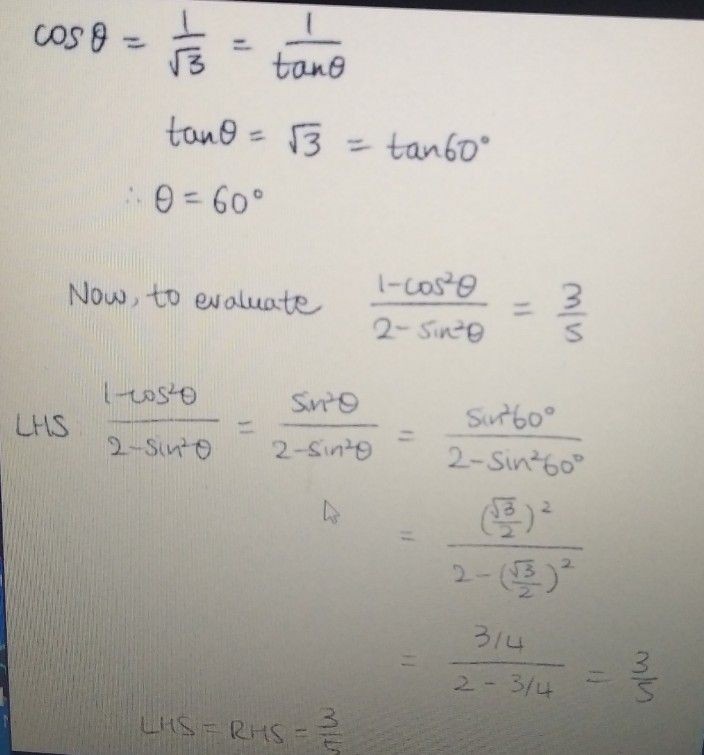Symbol
Problem$1$ If $cosθ=-$ $\sqrt{3}$ then evaluate $1-cos^{2}θ$ $3$ $-=-$ $2-sin^{2}θ$ $5$ $2.$ $3$ Evaluate $\int$ $|x^{2}-2x|dx$ $1$
Algebra
Search count: 173
SolutionQanda teacher - dharmender